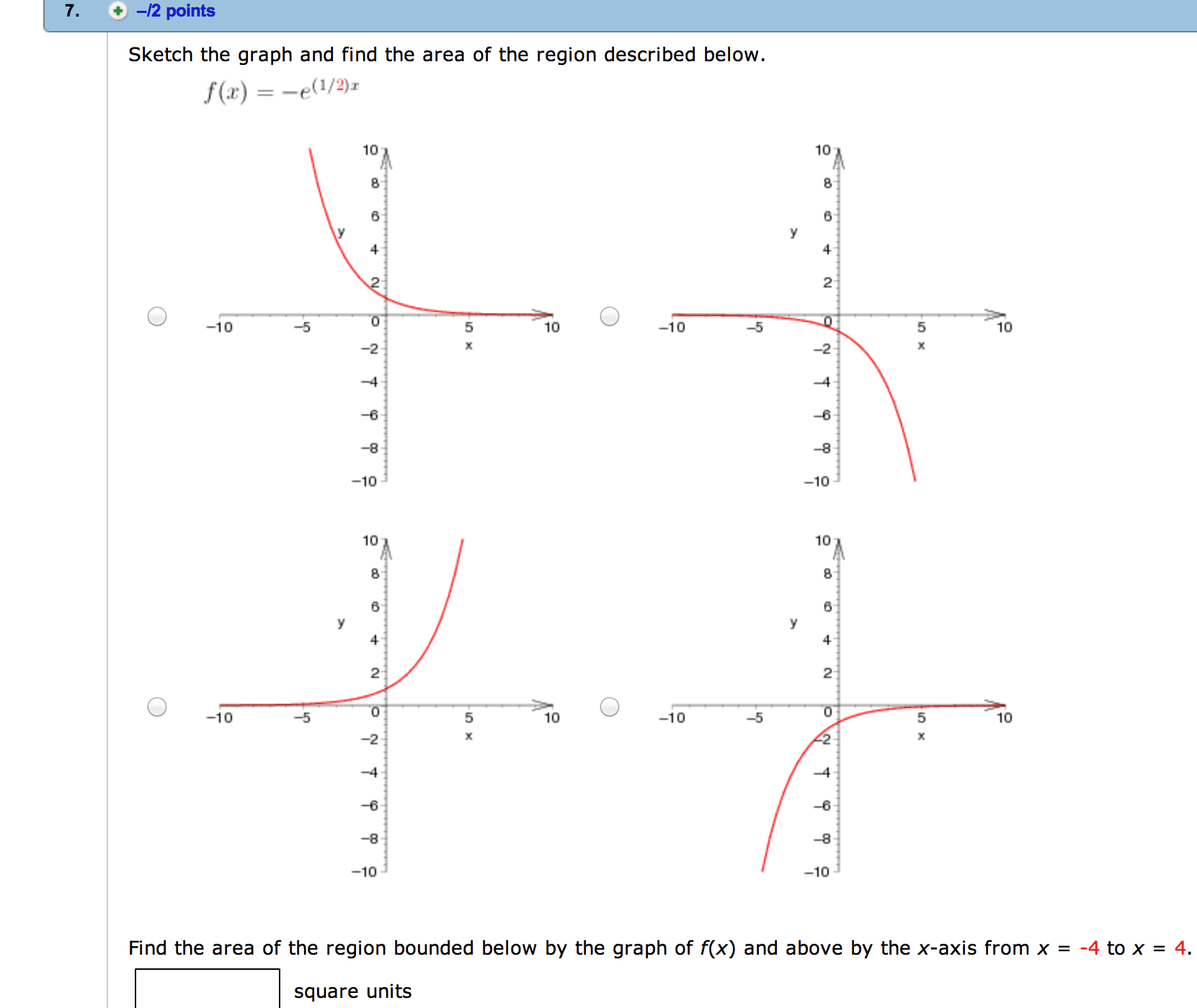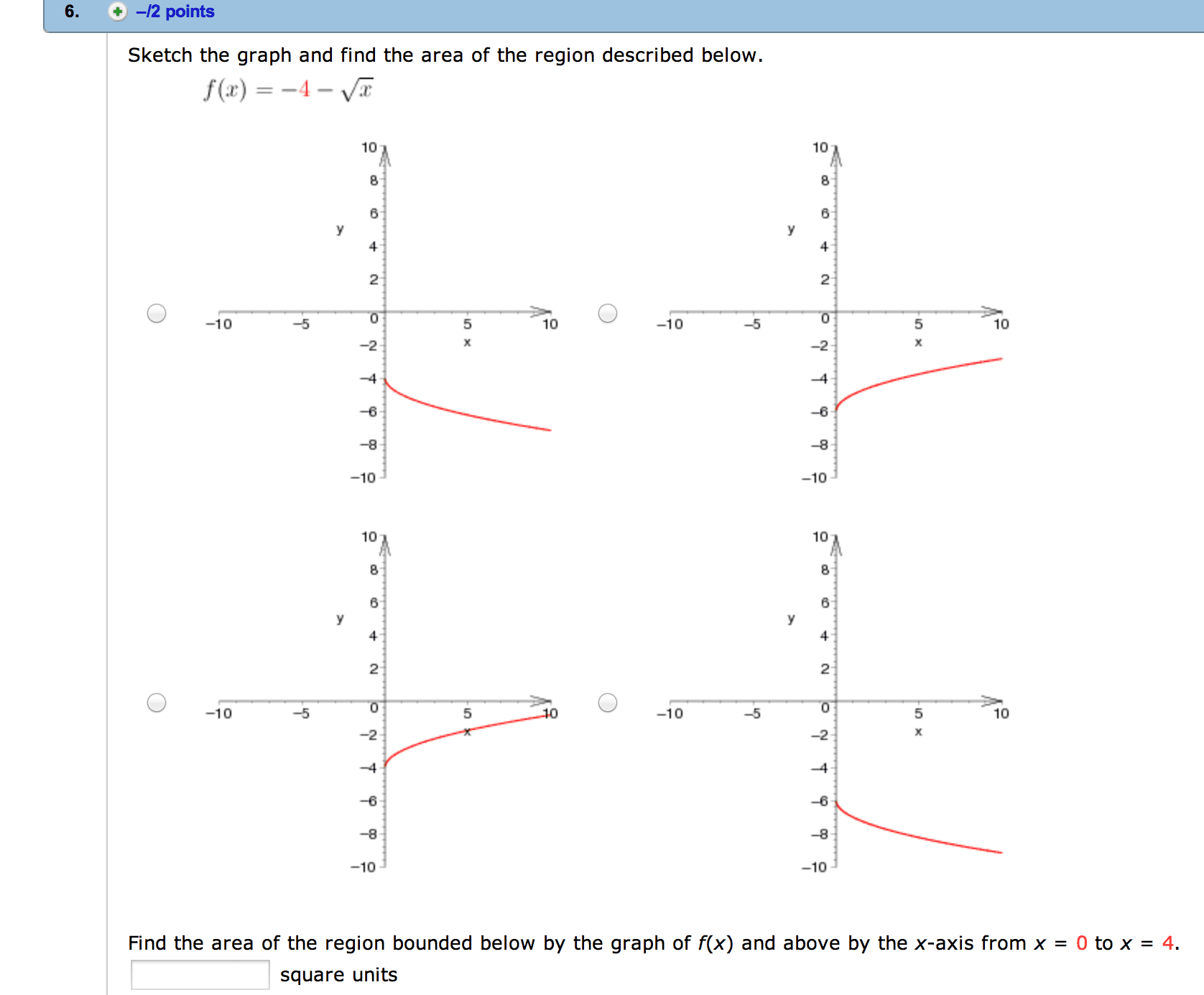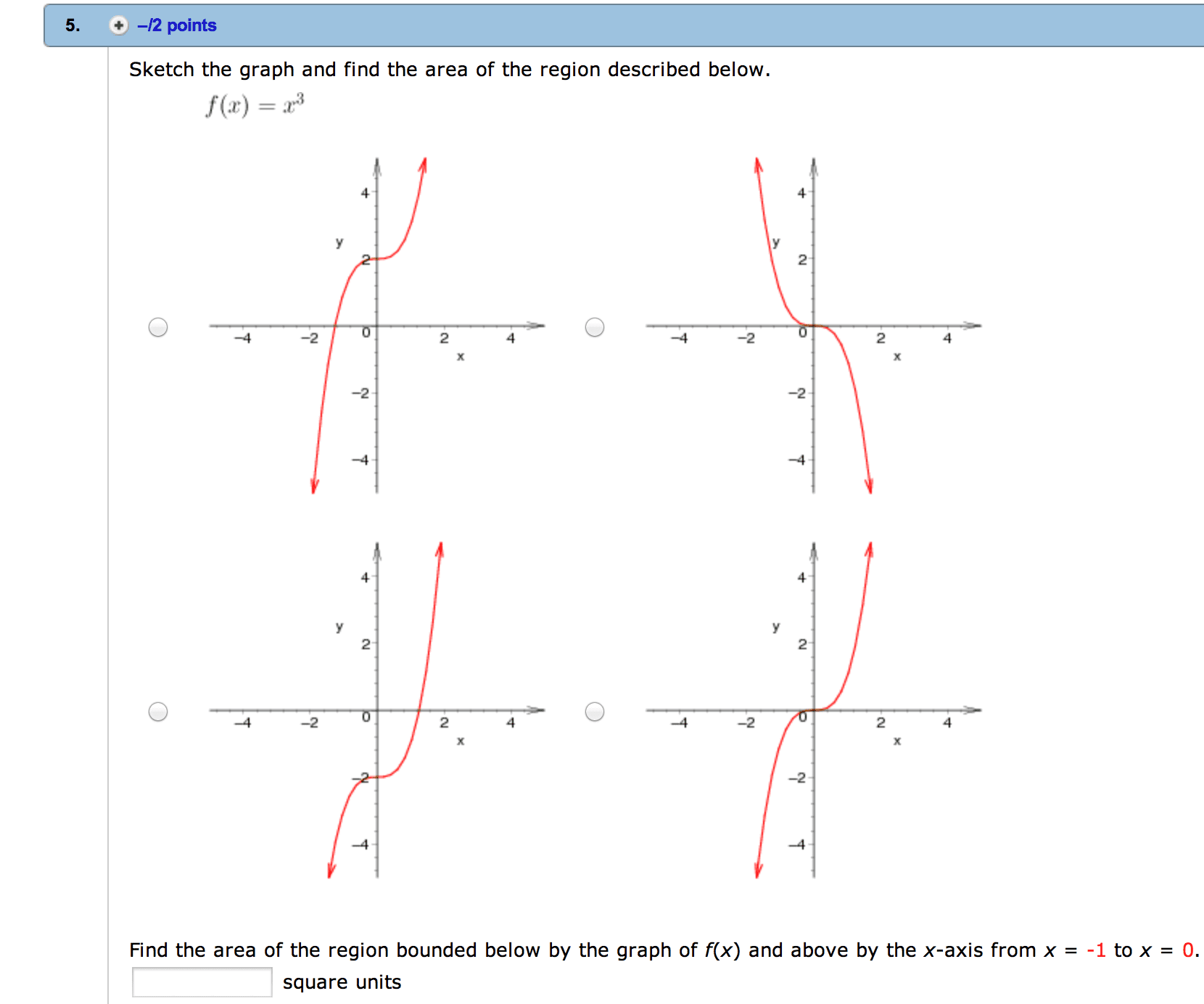1
0
watching
53
views

# Please answer:7. /2 points Sketch the graph and find the area of the region described below. (1/2)r f(a) 100 100 10 10 10 10 10 10 10 10 10 -5 10 10 10 -2 -8 10 10 Find the area of the region bounded below by the graph of f(x) and above by the x-axis from x -4 to x 4. square units Show transcribed image text 7. /2 points Sketch the graph and find the area of the region described below. (1/2)r f(a) 100 100 10 10 10 10 10 10 10 10 10 -5 10 10 10 -2 -8 10 10 Find the area of the region bounded below by the graph of f(x) and above by the x-axis from x -4 to x 4. square units